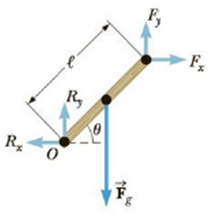Chapter 8, Problem 32P

Chapter
Section
Textbook Problem

Write the necessary equations of equilibrium of the object shown in Figure P8.32. Take the origin of the torque equation about an axis perpendicular to the page through the point O.33.The chewing muscle, the masseter, is one of the stron-To determine
The necessary equations of equilibrium of the object.

Explanation
For the object to be in equilibrium, the net torque about the point O is τ=0 and it is represented as Fy(lcosθ)Fx(lsinθ)Fg(l2cosθ)=0 This is possible only when Fx

Still sussing out bartleby?

Check out a sample textbook solution.

See a sample solution

The Solution to Your Study Problems

Bartleby provides explanations to thousands of textbook problems written by our experts, many with advanced degrees!

Get Started

Find more solutions based on key concepts